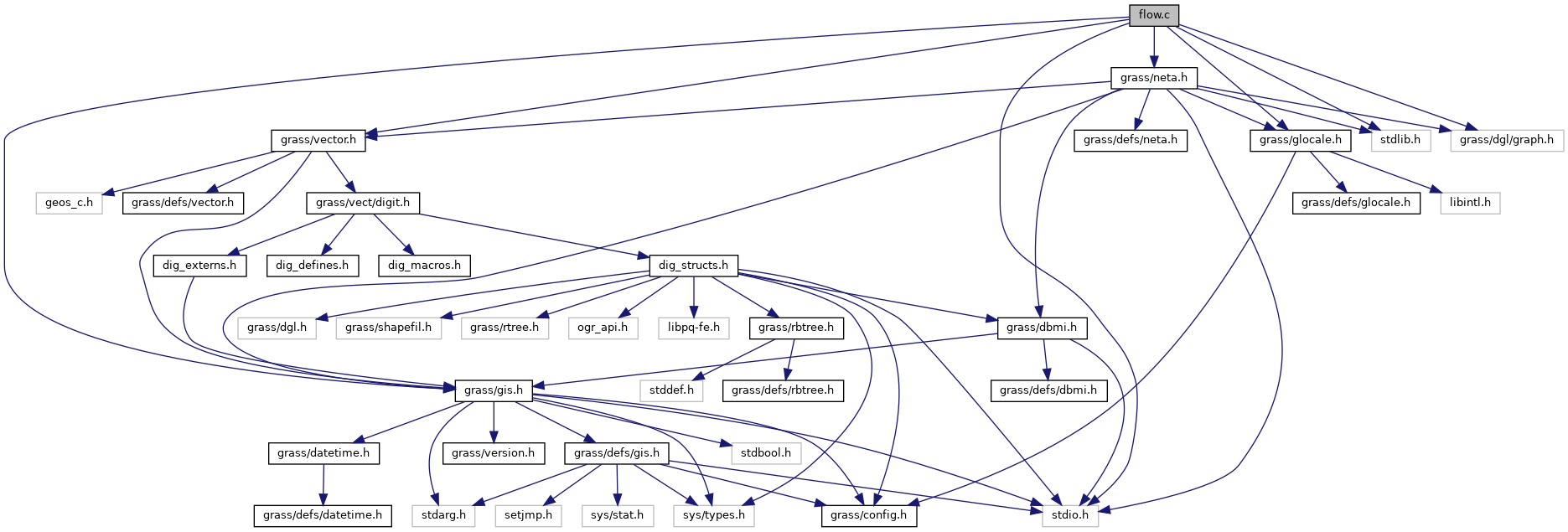GRASS GIS 7 Programmer's Manual  7.9.dev(2021)-e5379bbd7
flow.c File Reference

Network Analysis library - flow in graph. More...

`#include <stdio.h>`
`#include <stdlib.h>`
`#include <grass/gis.h>`
`#include <grass/vector.h>`
`#include <grass/glocale.h>`
`#include <grass/dgl/graph.h>`
`#include <grass/neta.h>`
Include dependency graph for flow.c:Go to the source code of this file.

## Functions

dglInt32_t sign (dglInt32_t x)

int NetA_flow (dglGraph_s *graph, struct ilist *source_list, struct ilist *sink_list, int *flow)
Get max flow from source to sink. More...

int NetA_min_cut (dglGraph_s *graph, struct ilist *source_list, struct ilist *sink_list, int *flow, struct ilist *cut)
Calculates minimum cut between source(s) and sink(s). More...

int NetA_split_vertices (dglGraph_s *in, dglGraph_s *out, int *node_costs)
Splits each vertex of in graph into two vertices. More...

## Detailed Description

Network Analysis library - flow in graph.

Computes the length of the shortest path between all pairs of nodes in the network.

(C) 2009-2010 by Daniel Bundala, and the GRASS Development Team

This program is free software under the GNU General Public License (>=v2). Read the file COPYING that comes with GRASS for details.

Definition in file flow.c.

## ◆ NetA_flow()

 int NetA_flow ( dglGraph_s * graph, struct ilist * source_list, struct ilist * sink_list, int * flow )

Get max flow from source to sink.

Array flow stores flow for each edge. Negative flow corresponds to a flow in opposite direction. The function assumes that the edge costs correspond to edge capacities.

Parameters
 graph input graph source_list list of sources sink_list list of sinks [out] flow max flows
Returns
number of flows
-1 on failure

Definition at line 47 of file flow.c.

## ◆ NetA_min_cut()

 int NetA_min_cut ( dglGraph_s * graph, struct ilist * source_list, struct ilist * sink_list, int * flow, struct ilist * cut )

Calculates minimum cut between source(s) and sink(s).

Flow is the array produced by NetA_flow() method when called with source_list and sink_list as the input. The output of this and NetA_flow() method should be the same.

Parameters
 graph input graph source_list list of sources sink_list list of sinks flow [out] cut list of edges (cut)
Returns
number of edges
-1 on failure

Definition at line 177 of file flow.c.

References _, dglGet_NodeCount(), G_calloc, G_fatal_error(), ilist::n_values, and ilist::value.

## ◆ NetA_split_vertices()

 int NetA_split_vertices ( dglGraph_s * in, dglGraph_s * out, int * node_costs )

Splits each vertex of in graph into two vertices.

The method splits each vertex of in graph into two vertices: in vertex and out vertex. Also, it adds an edge from an in vertex to the corresponding out vertex (capacity=2) and it adds an edge from out vertex to in vertex for each edge present in the in graph (forward capacity=1, backward capacity=0). If the id of a vertex is v then id of in vertex is 2*v-1 and of out vertex 2*v.

Parameters
 in from graph out to graph node_costs list of node costs
Returns
number of undirected edges in the graph
-1 on failure

Definition at line 270 of file flow.c.

## ◆ sign()

 dglInt32_t sign ( dglInt32_t x )

Definition at line 25 of file flow.c.

Referenced by Rast3d_fpcompress_dissect_xdr_double().